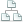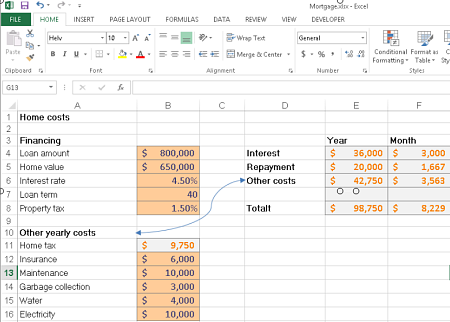﻿ Excel Tips from kalmstrom.com Business Solutions. Learn how to use Excel Formulas
 Home >Tips >Home costs calculationSite map

Use Formulas in Excel – multiply and divide

An Excel tutorial by Peter KalmstromThe possibility to tell Excel to calculate is a crucial feature and the most important reason to use it, and in an earlier demo we have seen how to create formulas that make Excel count plus and minus. In the demo below Peter Kalmstrom explains how to make Excel multiply and divide.

When you use a formula Excel calculates with the values of the cells you have given in the formula and gives the result in a separate cell. When the values in the cells are changed the figure in the result cell will change automatically.Excel is used for all kinds of calculations, from personal budgets to very advanced prognoses, and as an example for his demo Peter has taken the yearly and monthly costs for owning a home.

Peter shows how to:
• make Excel multiply the values of two cells
• make Excel divide values of two cells
• calculate sums
• use the function wizard
•  copy formulas
• change number format
• copy a format
• apply cell styles.
Peter uses Excel 2013 for his demo, but the formulas are the same for earlier versions of Excel.